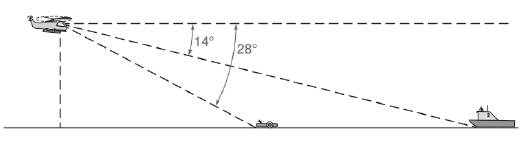Chapter 11.3, Problem 44EElementary Geometry For College St...

7th Edition
Alexander + 2 others
ISBN: 9781337614085

Solutions

Chapter
SectionElementary Geometry For College St...

7th Edition
Alexander + 2 others
ISBN: 9781337614085
Textbook Problem

In Exercises 39 to 45, angle measures should be given to the nearest degree; distance should be given to the nearest tenth of a unit.While a helicopter hovers 1000 ft above the water, its pilot spies a man in a lifeboat through an angle of depression of 28 ∘ . Along a straight line, a rescue boat can also be seen through an angle depression of 14 ∘ . How far is the rescue boat from the lifeboat?To determine

To calculate: The distance between the rescue boat from the lifeboat.

Explanation

Given:

While a helicopter hovers 100ft above the water, its pilot spies a man in a lifeboat through an angle of depression of 28. Along a straight line, a rescue boat can also seen through an angle of depression of 14.

Calculation:

Now, we have to find the value of BE.

Still sussing out bartleby?

Check out a sample textbook solution.

See a sample solution

The Solution to Your Study Problems

Bartleby provides explanations to thousands of textbook problems written by our experts, many with advanced degrees!

Get Started

Find the mean, median, and mode for the following scores; 8, 7, 5, 7, 0, 10, 2, 4, 11, 7, 8, 7

Essentials of Statistics for The Behavioral Sciences (MindTap Course List)

Express 58 as a decimal.

Elementary Technical Mathematics

Differentiate the function. f(x) = x ln x x

Single Variable Calculus: Early Transcendentals

Rectangular coordinates of the point with polar coordinates are: (−1, 0) (0, 1) (0, −1) (1, 0)

Study Guide for Stewart's Single Variable Calculus: Early Transcendentals, 8th

In Exercises 57-62, Use Venn diagram to illustrate each statement. A(BC)=(AB)(AC)

Finite Mathematics for the Managerial, Life, and Social Sciences